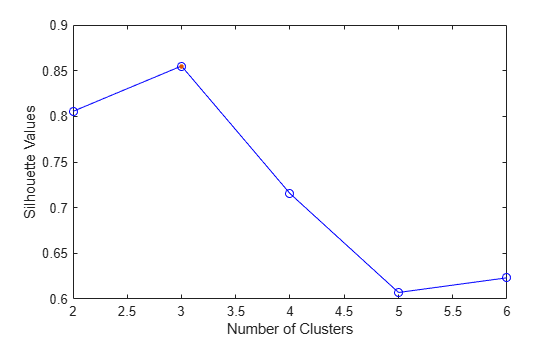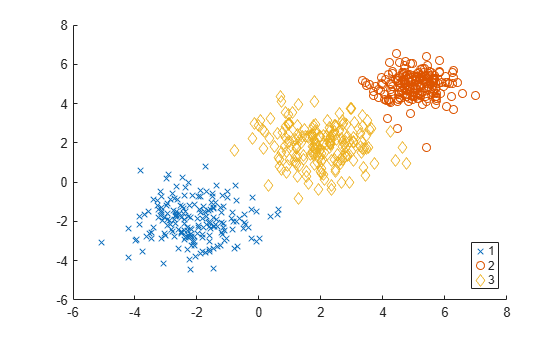# SilhouetteEvaluation

Package: clustering.evaluation
Superclasses: `clustering.evaluation.ClusterCriterion`

Silhouette criterion clustering evaluation object

## Description

`SilhouetteEvaluation` is an object consisting of sample data, clustering data, and silhouette criterion values used to evaluate the optimal number of data clusters. Create a silhouette criterion clustering evaluation object using `evalclusters`.

## Construction

`eva = evalclusters(x,clust,'Silhouette')` creates a silhouette criterion clustering evaluation object.

`eva = evalclusters(x,clust,'Silhouette',Name,Value)` creates a silhouette criterion clustering evaluation object using additional options specified by one or more name-value pair arguments.

### Input Arguments

expand all

Input data, specified as an N-by-P matrix. N is the number of observations, and P is the number of variables.

Data Types: `single` | `double`

Clustering algorithm, specified as one of the following.

 `'kmeans'` Cluster the data in `x` using the `kmeans` clustering algorithm, with `'EmptyAction'` set to `'singleton'` and `'Replicates'` set to `5`. `'linkage'` Cluster the data in `x` using the `clusterdata` agglomerative clustering algorithm, with `'Linkage'` set to `'ward'`. `'gmdistribution'` Cluster the data in `x` using the `gmdistribution` Gaussian mixture distribution algorithm, with `'SharedCov'` set to `true` and `'Replicates'` set to `5`.

If `criterion` is `'CalinskiHarabasz'`, `'DaviesBouldin'`, or `'silhouette'`, you can specify a clustering algorithm using a function handle (MATLAB). The function must be of the form `C = clustfun(DATA,K)`, where `DATA` is the data to be clustered, and `K` is the number of clusters. The output of `clustfun` must be one of the following:

• A vector of integers representing the cluster index for each observation in `DATA`. There must be `K` unique values in this vector.

• A numeric n-by-K matrix of score for n observations and K classes. In this case, the cluster index for each observation is determined by taking the largest score value in each row.

If `criterion` is `'CalinskiHarabasz'`, `'DaviesBouldin'`, or `'silhouette'`, you can also specify `clust` as a n-by-K matrix containing the proposed clustering solutions. n is the number of observations in the sample data, and K is the number of proposed clustering solutions. Column j contains the cluster indices for each of the N points in the jth clustering solution.

Data Types: `single` | `double` | `char` | `string` | `function_handle`

#### Name-Value Pair Arguments

Specify optional comma-separated pairs of `Name,Value` arguments. `Name` is the argument name and `Value` is the corresponding value. `Name` must appear inside quotes. You can specify several name and value pair arguments in any order as `Name1,Value1,...,NameN,ValueN`.

Example: `'KList',[1:5],'Distance','cityblock'` specifies to test 1, 2, 3, 4, and 5 clusters using the city block distance metric.

Prior probabilities for each cluster, specified as the comma-separated pair consisting of `'ClusterPriors'` and one of the following.

 `'empirical'` Compute the overall silhouette value for the clustering solution by averaging the silhouette values for all points. Each cluster contributes to the overall silhouette value proportionally to its size. `'equal'` Compute the overall silhouette value for the clustering solution by averaging the silhouette values for all points within each cluster, and then averaging those values across all clusters. Each cluster contributes equally to the overall silhouette value, regardless of its size.

Example: `'ClusterPriors','empirical'`

Distance metric used for computing the criterion values, specified as the comma-separated pair consisting of `'Distance'` and one of the following.

 `'sqEuclidean'` Squared Euclidean distance `'Euclidean'` Euclidean distance. This option is not valid for the `kmeans` clustering algorithm. `'cityblock'` Sum of absolute differences `'cosine'` One minus the cosine of the included angle between points (treated as vectors) `'correlation'` One minus the sample correlation between points (treated as sequences of values) `'Hamming'` Percentage of coordinates that differ. This option is only valid for the `Silhouette` criterion. `'Jaccard'` Percentage of nonzero coordinates that differ. This option is only valid for the `Silhouette` criterion.

For detailed information about each distance metric, see `pdist`.

You can also specify a function for the distance metric using a function handle (MATLAB). The distance function must be of the form `d2 = distfun(XI,XJ)`, where `XI` is a 1-by-n vector corresponding to a single row of the input matrix `X`, and `XJ` is an m2-by-n matrix corresponding to multiple rows of `X`. `distfun` must return an m2-by-1 vector of distances `d2`, whose kth element is the distance between `XI` and `XJ(k,:)`.

`Distance` only accepts a function handle if the clustering algorithm `clust` accepts a function handle as the distance metric. For example, the `kmeans` clustering algorithm does not accept a function handle as the distance metric. Therefore, if you use the `kmeans` algorithm and then specify a function handle for `Distance`, the software errors.

• If `criterion` is `'silhouette'`, you can also specify `Distance` as the output vector created by the function `pdist`.

• When `clust` is `'kmeans'` or `'gmdistribution'`, `evalclusters` uses the distance metric specified for `Distance` to cluster the data.

• If `clust` is `'linkage'`, and `Distance` is either `'sqEuclidean'` or `'Euclidean'`, then the clustering algorithm uses the Euclidean distance and Ward linkage.

• If `clust` is `'linkage'` and `Distance` is any other metric, then the clustering algorithm uses the specified distance metric and average linkage.

• In all other cases, the distance metric specified for `Distance` must match the distance metric used in the clustering algorithm to obtain meaningful results.

Example: `'Distance','Euclidean'`

Data Types: `single` | `double` | `char` | `string` | `function_handle`

List of number of clusters to evaluate, specified as the comma-separated pair consisting of `'KList'` and a vector of positive integer values. You must specify `KList` when `clust` is a clustering algorithm name or a function handle. When `criterion` is `'gap'`, `clust` must be a character vector, a string scalar, or a function handle, and you must specify `KList`.

Example: `'KList',[1:6]`

Data Types: `single` | `double`

## Properties

 `ClusteringFunction` Clustering algorithm used to cluster the input data, stored as a valid clustering algorithm name or function handle. If the clustering solutions are provided in the input, `ClusteringFunction` is empty. `ClusterPriors` Prior probabilities for each cluster, stored as valid prior probability name. `ClusterSilhouettes` Silhouette values corresponding to each proposed number of clusters in `InspectedK`, stored as a cell array of vectors. `CriterionName` Name of the criterion used for clustering evaluation, stored as a valid criterion name. `CriterionValues` Criterion values corresponding to each proposed number of clusters in `InspectedK`, stored as a vector of numerical values. `Distance` Distance metric used for clustering data, stored as a valid distance metric name. `InspectedK` List of the number of proposed clusters for which to compute criterion values, stored as a vector of positive integer values. `Missing` Logical flag for excluded data, stored as a column vector of logical values. If `Missing` equals `true`, then the corresponding value in the data matrix `x` is not used in the clustering solution. `NumObservations` Number of observations in the data matrix `X`, minus the number of missing (`NaN`) values in `X`, stored as a positive integer value. `OptimalK` Optimal number of clusters, stored as a positive integer value. `OptimalY` Optimal clustering solution corresponding to `OptimalK`, stored as a column vector of positive integer values. If the clustering solutions are provided in the input, `OptimalY` is empty. `X` Data used for clustering, stored as a matrix of numerical values.

## Methods

### Inherited Methods

 addK Evaluate additional numbers of clusters compact Compact clustering evaluation object plot Plot clustering evaluation object criterion values

## Examples

collapse all

Evaluate the optimal number of clusters using the silhouette clustering evaluation criterion.

Generate sample data containing random numbers from three multivariate distributions with different parameter values.

```rng('default'); % For reproducibility mu1 = [2 2]; sigma1 = [0.9 -0.0255; -0.0255 0.9]; mu2 = [5 5]; sigma2 = [0.5 0 ; 0 0.3]; mu3 = [-2, -2]; sigma3 = [1 0 ; 0 0.9]; N = 200; X = [mvnrnd(mu1,sigma1,N);... mvnrnd(mu2,sigma2,N);... mvnrnd(mu3,sigma3,N)];```

Evaluate the optimal number of clusters using the silhouette criterion. Cluster the data using `kmeans`.

`E = evalclusters(X,'kmeans','silhouette','klist',[1:6])`
```E = SilhouetteEvaluation with properties: NumObservations: 600 InspectedK: [1 2 3 4 5 6] CriterionValues: [NaN 0.8055 0.8551 0.7155 0.6071 0.6232] OptimalK: 3 ```

The `OptimalK` value indicates that, based on the silhouette criterion, the optimal number of clusters is three.

Plot the silhouette criterion values for each number of clusters tested.

```figure; plot(E)```The plot shows that the highest silhouette value occurs at three clusters, suggesting that the optimal number of clusters is three.

Create a grouped scatter plot to visually examine the suggested clusters.

```figure; gscatter(X(:,1),X(:,2),E.OptimalY,'rbg','xod')```The plot shows three distinct clusters within the data: Cluster 1 is in the lower-left corner, cluster 2 is in the upper-right corner, and cluster 3 is near the center of the plot.

expand all

## References

 Kaufman L. and P. J. Rouseeuw. Finding Groups in Data: An Introduction to Cluster Analysis. Hoboken, NJ: John Wiley & Sons, Inc., 1990.

 Rouseeuw, P. J. “Silhouettes: a graphical aid to the interpretation and validation of cluster analysis.” Journal of Computational and Applied Mathematics. Vol. 20, No. 1, 1987, pp. 53–65.